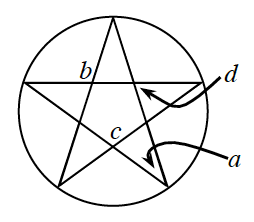### Home > CCG > Chapter 12 > Lesson 12.1.2 > Problem12-22

12-22.The figure at right is a pentagram. A pentagram is a $5$-pointed star that has congruent angles at each of its outer vertices.

1. Use the fact that all pentagrams can be inscribed in a circle to find the measure of angle $a$ at right.

Find the measure of each of the intercepted arcs.

$\frac{360^\circ}{5} = 72^\circ$

The degree measure of an inscribed angle is half the measure of the intercepted arc.

$\frac{72^\circ}{2}$

$=36^\circ$

2. Find the measure of angles $b$, $c$, and $d$.

Use the information found in part (a) and remember that a pentagram has a regular pentagon inside of it, and the angles in a regular pentagon are equal.